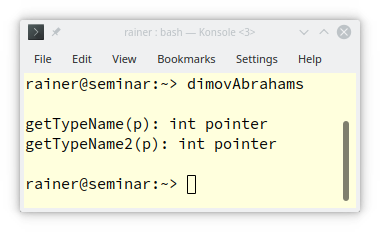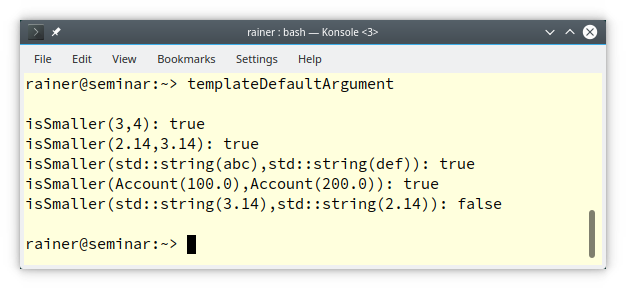# Full Specialization of Function Templates

As you may know from my previous post, Template Specialization, a function template can only be full but not partially specialized. To make my long story short: Don’t specialize function templates. Just use function overloading.You may wonder why I write about a feature of C++ you should not use. The reason is quite simple. When you see the surprising behavior of fully specialized function templates, you will hopefully use a non-generic function instead.

## Don’t Specialize Function Templates

Maybe the title reminds you? Right. This title is from the C++ Core Guidelines: T.144: Don’t specialize function templates

The reason for the rules is relatively short: function template specialization doesn’t participate in overloading. Let’s see what that means. My program is based on the program snippet from Dimov/Abrahams.

```// dimovAbrahams.cpp

#include <iostream>
#include <string>

// getTypeName

template<typename T>            // (1) primary template
std::string getTypeName(T){
return "unknown";
}

template<typename T>            // (2) primary template that overloads (1)
std::string getTypeName(T*){
return "pointer";
}

template<>                      // (3) explicit specialization of (2)
std::string getTypeName(int*){
return "int pointer";
}

// getTypeName2

template<typename T>            // (4) primary template
std::string getTypeName2(T){
return "unknown";
}

template<>                      // (5) explicit specialization of (4)
std::string getTypeName2(int*){
return "int pointer";
}

template<typename T>            // (6) primary template that overloads (4)
std::string getTypeName2(T*){
return "pointer";
}

int main(){

std::cout << '\n';

int* p;

std::cout << "getTypeName(p): " << getTypeName(p) <<  '\n';
std::cout << "getTypeName2(p): " << getTypeName2(p) <<  '\n';

std::cout <<  '\n';

}
```

Admittedly, the code looks quite boring but bear with me. I defined inline (1) the primary template getTypeName. (2) is an overload for pointers, and (3) a full specialization for an `int` pointer. In the case of getTypeName2, I made a small variation. I put the explicit `specialisation` (5) before the overload for pointers (6).

##Modernes C++ Mentoring

Be part of my mentoring programs:

• "Fundamentals for C++ Professionals" (open)
• "Design Patterns and Architectural Patterns with C++" (open)
• "C++20: Get the Details" (reopens December 2023)
• Do you want to stay informed about my mentoring programs: Subscribe via E-Mail.

This reordering has surprising consequences.In the first case, the full specialization for the int pointer is called, and in the second case, the overload of pointers. What?  This non-intuitive behavior is because overload resolution ignores function template specialization. Overload resolution operates on primary templates and functions. In both cases, overload resolutions found both primary templates. In the first case (getTypeName), the pointer variant is the better fit and, therefore, the explicit specialization for the int pointer was chosen. The pointer variant was chosen in the second variant (getTypeName2), but the full specialization belongs to the primary template (line 4). Consequently, it was ignored.

I know this wasn’t very easy. Just keep the rule in mind: Don’t specialize function templates; use non-generic functions instead.

Do you want to have proof of my statement? Here it is: Making out of the explicit specialization in (3) and (5) non-generic functions solves the issue. I have to comment out the template declaration` template<>`. For simplicity reasons, I removed the other comments.

```// dimovAbrahams.cpp

#include <iostream>
#include <string>

// getTypeName

template<typename T>
std::string getTypeName(T){
return "unknown";
}

template<typename T>
std::string getTypeName(T*){
return "pointer";
}
// template<>                      // (3)
std::string getTypeName(int*){
return "int pointer";
}

// getTypeName2

template<typename T>
std::string getTypeName2(T){
return "unknown";
}
// template<>                      // (5)
std::string getTypeName2(int*){
return "int pointer";
}

template<typename T>
std::string getTypeName2(T*){
return "pointer";
}

int main(){

std::cout << '\n';

int* p;

std::cout << "getTypeName(p): " << getTypeName(p) <<  '\n';
std::cout << "getTypeName2(p): " << getTypeName2(p) <<  '\n';

std::cout <<  '\n';

}
```

Now, function overloading works as expected, and the non-generic function takes an `int` pointer is used.I already wrote about Template Arguments. But I forgot one crucial fact. You can provide default template arguments for function templates and class templates.

## Default Template Arguments

What is common to the Standard Template Library class templates (STL)? Yes! Many of the template arguments have defaults.

Here are a few examples.

```template<
typename T,
typename Allocator = std::allocator<T>
> class vector;

template<
typename Key,
typename T,
typename Hash = std::hash<Key>,
typename KeyEqual = std::equal_to<Key>,
typename Allocator = std::allocator< std::pair<const Key, T>>
> class unordered_map;

template<
typename T,
typename Allocator = std::allocator<T>
> class deque;

template<
typename T,
typename Container = std::deque<T>
> class stack;

template<
typename CharT,
typename Traits = std::char_traits<CharT>,
typename Allocator = std::allocator<CharT>
> class basic_string;
```

This is part of the power of the STL:

• Each container has a default allocator that depends on its elements.
• You have to specify the required arguments, such as the essential type and value type, for a `std::unordered_map: std::unordered_map<std::string, int>. `
• You can instantiate a` std::unordered_map` using a particular hash function returning the has value for the key and a unique binary predicate determining if two keys are equal: `std::unordered_map<std::string, int, MyHash>`, or `std::unordered_map<std::string, int, MyHash, MyBinaryPredicate>`.

• std::string is just an alias for common character types. Here are the aliases based on` std::basic_string.`

```std::string         std::basic_string<char>
std::wstring 	    std::basic_string<wchar_t>
std::u8string 	    std::basic_string<char8_t>  (C++20)
std::u16string      std::basic_string<char16_t> (C++11)
std::u32string      std::basic_string<char32_t> (C++11)
```

Of course, when a template argument has a default, the following template arguments must also have a default.

So far, I only wrote about default template arguments for class templates. I want to end this post with an example of function templates.

Assume I want to decide for a few objects having the same type which one is smaller. An algorithm such as `isSmaller` models a generic idea and should, therefore, be a template.

```// templateDefaultArguments.cpp

#include <functional>
#include <iostream>
#include <string>

class Account{
public:
explicit Account(double b): balance(b){}
double getBalance() const {
return balance;
}
private:
double balance;
};

template <typename T, typename Pred = std::less<T>>                        // (1)
bool isSmaller(T fir, T sec, Pred pred = Pred() ){
return pred(fir,sec);
}

int main(){

std::cout << std::boolalpha << '\n';

std::cout << "isSmaller(3,4): " << isSmaller(3,4) << '\n';                // (2)
std::cout << "isSmaller(2.14,3.14): "  << isSmaller(2.14,3.14) << '\n';
std::cout << "isSmaller(std::string(abc),std::string(def)): " <<
isSmaller(std::string("abc"),std::string("def")) << '\n';

bool resAcc= isSmaller(Account(100.0),Account(200.0),                     // (3)
[](const Account& fir, const Account& sec){ return fir.getBalance() < sec.getBalance(); });
std::cout << "isSmaller(Account(100.0),Account(200.0)): " << resAcc << '\n';

bool acc= isSmaller(std::string("3.14"),std::string("2.14"),              // (4)
[](const std::string& fir, const std::string& sec){ return std::stod(fir) < std::stod(sec); });
std::cout << "isSmaller(std::string(3.14),std::string(2.14)): " << acc << '\n';

std::cout << '\n';

}
```

In the default case (2), `isSmaller` works as expected. `isSmaller` (1) uses the template argument `std::less` , one of many predefined function objects in the STL. It applies the less-than operator `<` to its arguments. To use it, I had to instantiate std::less<T> in the following line:` Pred pred = Pred()`.

I can compare accounts (3) or strings (4) thanks to the default template argument. `Account` does not support the less-than-operator. Nevertheless, I can compare `Account`s. (3). Additionally, I want to compare strings not lexicographically but based on their internal number (4). Providing the two lambda expressions in (3) and (4) as binary predicates let me do my job successfully.## What’s next?

When you study the graphic at the beginning of this post, you see that I’m done with the basics of templates. In my next post about templates, I will dive further into the details and write about template instantiation.

Thanks a lot to my Patreon Supporters: Matt Braun, Roman Postanciuc, Tobias Zindl, G Prvulovic, Reinhold Dröge, Abernitzke, Frank Grimm, Sakib, Broeserl, António Pina, Sergey Agafyin, Андрей Бурмистров, Jake, GS, Lawton Shoemake, Jozo Leko, John Breland, Venkat Nandam, Jose Francisco, Douglas Tinkham, Kuchlong Kuchlong, Robert Blanch, Truels Wissneth, Kris Kafka, Mario Luoni, Friedrich Huber, lennonli, Pramod Tikare Muralidhara, Peter Ware, Daniel Hufschläger, Alessandro Pezzato, Bob Perry, Satish Vangipuram, Andi Ireland, Richard Ohnemus, Michael Dunsky, Leo Goodstadt, John Wiederhirn, Yacob Cohen-Arazi, Florian Tischler, Robin Furness, Michael Young, Holger Detering, Bernd Mühlhaus, Matthieu Bolt, Stephen Kelley, Kyle Dean, Tusar Palauri, Dmitry Farberov, Juan Dent, George Liao, Daniel Ceperley, Jon T Hess, Stephen Totten, Wolfgang Fütterer, Matthias Grün, Phillip Diekmann, Ben Atakora, Ann Shatoff, Rob North, and Bhavith C Achar.

Thanks, in particular, to Jon Hess, Lakshman, Christian Wittenhorst, Sherhy Pyton, Dendi Suhubdy, Sudhakar Belagurusamy, Richard Sargeant, Rusty Fleming, John Nebel, Mipko, Alicja Kaminska, Slavko Radman, and David Poole.

 My special thanks to EmbarcaderoMy special thanks to PVS-StudioMy special thanks to Tipi.buildMy special thanks to Take Up Code## Seminars

I’m happy to give online seminars or face-to-face seminars worldwide. Please call me if you have any questions.

### Standard Seminars (English/German)

Here is a compilation of my standard seminars. These seminars are only meant to give you a first orientation.

• C++ – The Core Language
• C++ – The Standard Library
• C++ – Compact
• C++11 and C++14
• Concurrency with Modern C++
• Design Pattern and Architectural Pattern with C++
• Embedded Programming with Modern C++
• Generic Programming (Templates) with C++

#### New

• Clean Code with Modern C++
• C++20

### Contact Me

Modernes C++ Mentoring,0 replies